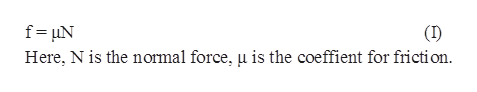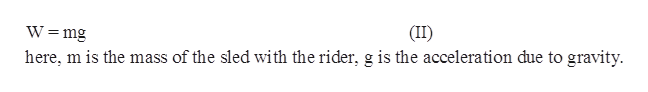A team of eight dogs pulls a sled with waxed wood runners on wet snow.  The dogs have average masses of 19.0 kg and the loaded sled with its rider has a mass of 210 kg.  Each dog exerts an average force of 185 N backwards on the snow.  What is the magnitude of the acceleration once the sled starts to move in m/s2?Group of answer choices2345

Question
A team of eight dogs pulls a sled with waxed wood runners on wet snow.  The dogs have average masses of 19.0 kg and the loaded sled with its rider has a mass of 210 kg.  Each dog exerts an average force of 185 N backwards on the snow.  What is the magnitude of the acceleration once the sled starts to move in m/s2?
Group of answer choices
2
3
4
5
Step 1

Given that mass of the sled and its rider is M=210kg,

Mass of each dog is m=19kg,

Number of dogs is n=8,

The force exerted by each dogs is f=185N

Step 2

The equation for magnitude of the friction force is.help_outlineImage Transcriptionclosef uN Here, N is the normal force, u is the coeffient for friction (I) fullscreen
Step 3

The equation for the weigh...help_outlineImage TranscriptioncloseW =mg (II) here, m is the mass of the sled with the rider, g is the acceleration due to gravity fullscreen

Want to see the full answer?

See Solution

Want to see this answer and more?

Our solutions are written by experts, many with advanced degrees, and available 24/7

See Solution
Tagged in

Physics